# 从 MLP 到 Self-Attention，一文总览用户行为序列推荐模型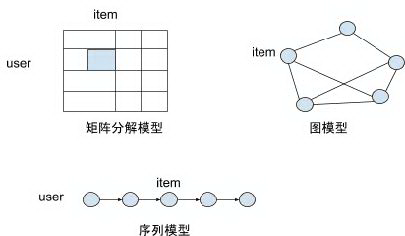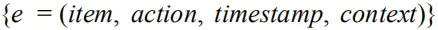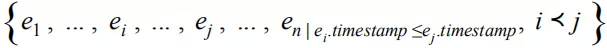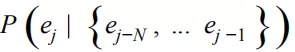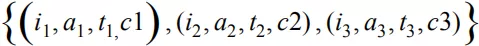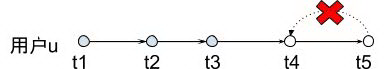## 数据准备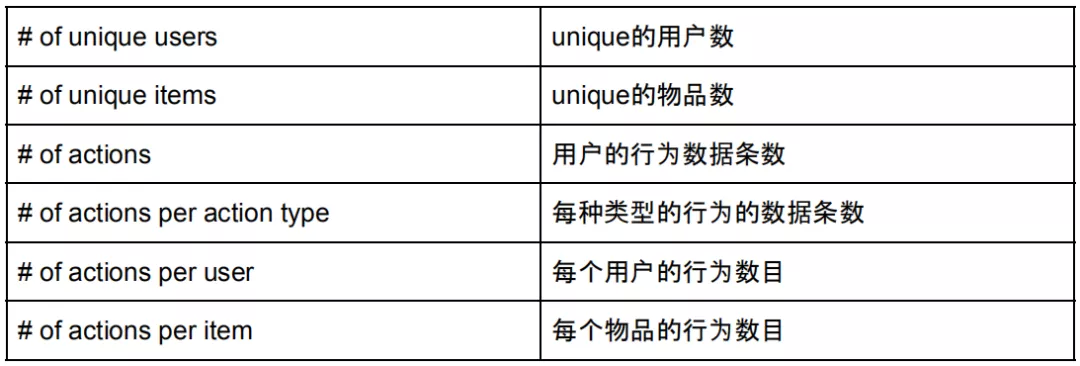❶ 无效用户过滤，如 robot ，网站爬虫等。这些用户的行为条目数通常远大于正常用户的行为数，可以结合用户行为数目的直方图，通过一些基于统计的异常点检测算法 ( 如检测，MAD- 基于绝对离差中位数，基于密度的检测方法等 ) 找到这些用户并过滤掉；

❷ 热门用户和热门物品的处理，可以对热门用户和物品对应的数据进行降采样，或者是进行降权，以减少热门度对推荐结果的影响；长序列可以根据时间进行切分，减少长序列对模型收敛性的影响。

## MLP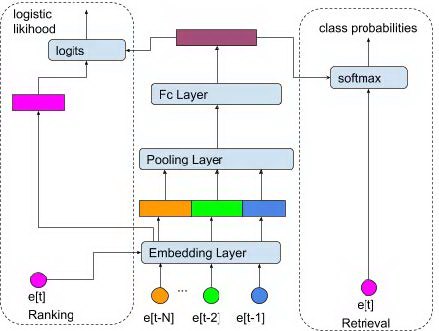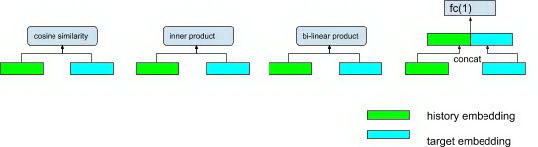• cosine similarity:$H\odot T/(|H|~|T|)$
• inner product:$H\odot T$
• bi-linear product:$(HW)\odot T$
• fully-connected layer:$[H,T]W+b$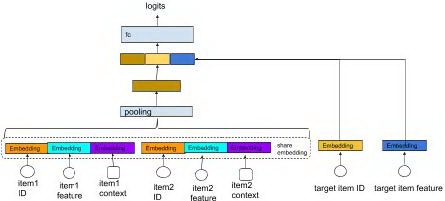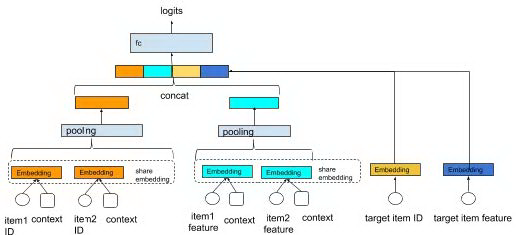Embedding 层

embedding 层的作用是：将序列中的事件信息映射为一个浮点数向量。每个事件的信息包括物品特征信息以及上下文信息，其中物品的特征包括离散型特征 ( 物品的 ID 编号，物品的类别，物品的标签等 ) 和连续型特征 ( 物品的历史热度等 )，其中物品的 ID 和类别是每个物品唯一标识，属于 univalent 离散特征，而每个物品包含的标签不止一个，为 multivalent 离散特征。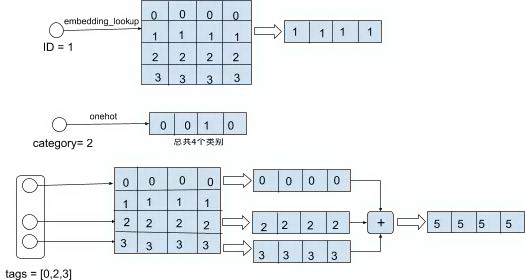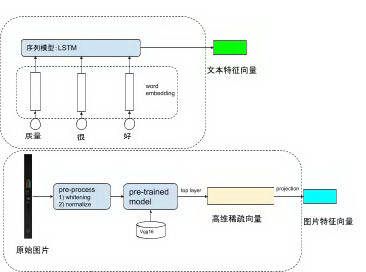$[0,1),[1,2),[2,4),\dots,[2^k,2^{k+1})$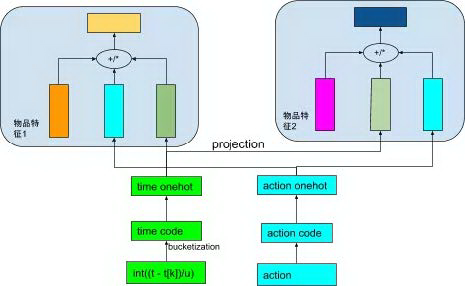Pooling 层

$SumPooling(e1,e2,\dots,eL;k)=\sum^L_{i=1}e_{i,k},1\leq k\leq d$

$AveragePooling(e1,e2,\dots,2L;k)=\sum^L_{i=1}e_{i,k}/L,1\leq k\leq d$

k 表示向量的第 k 维，d 为向量维度。

FC 层

FC 层相对比较简单：pooling 层的输出向量经过多个全连接层，最终输出用户对候选物品的兴趣的预估：P(y|x1,x2,…xn)。全连接层的输出维度一般是逐渐变小，一方面起到降维的作用，并且通过全连接去学习不同特征之间的高阶关联关系，全连接层采用非线性激活函数将所有特征向量单元之间的关联进行非线性化抽象，从而提升模型的描述能力。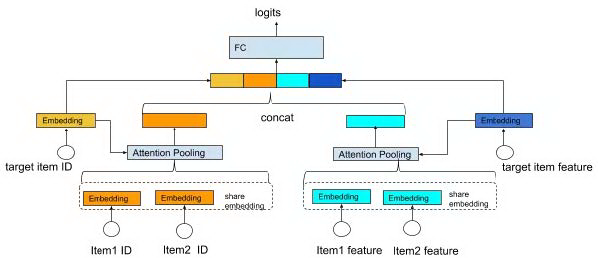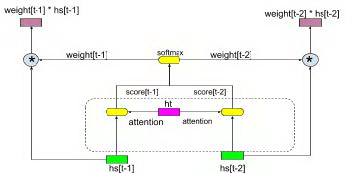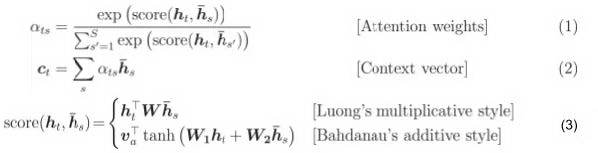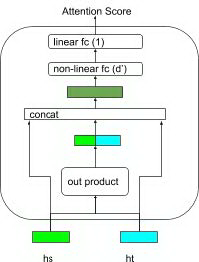## CNN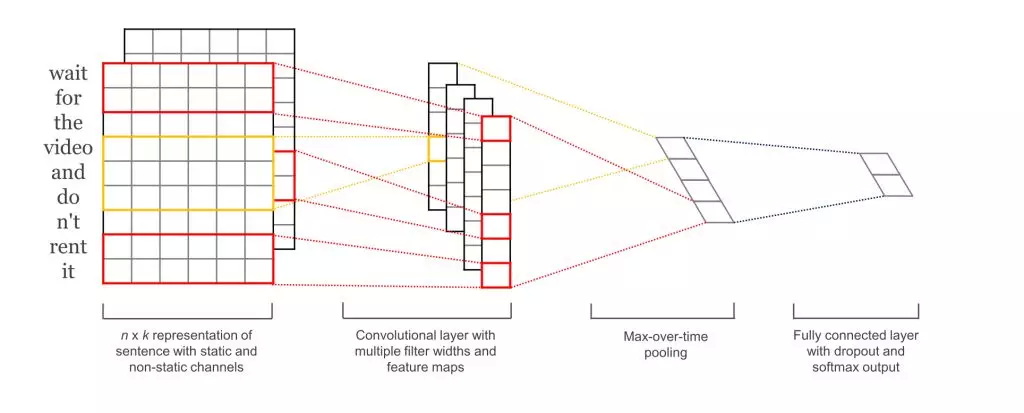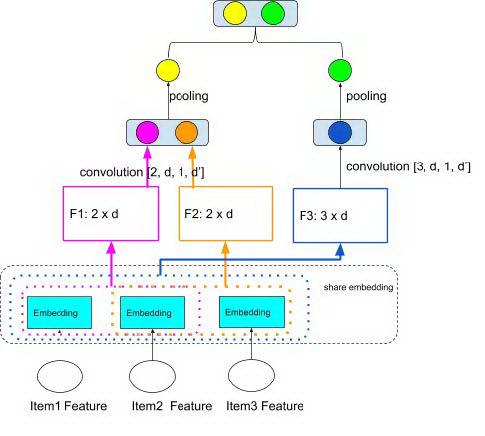pooling_outputs = []for kernel_size in kernels:     #使用一维卷积，相当于 [kernel_size, input_emb_size, 1, output_dimension] 二维卷积     conv_out = tf.layers.conv1d(input_emb, kernel_size, output_dimension, strides=1, padding=’VALID’)     #在序列长度维度进行最大值池化     #pool_out 的 shape 变为 [batch_size, output_dimension]     pool_out = tf.reduce_max(conv_out, -2)     pooling_outputs.append(pool_out)#所有池化生成的向量进行拼接return tf.concat(pooling_outputs, -1)

CNN 和 MLP 也可以结合在一起使用，在 pooling 层采用全局的 pooling 提取用户行为序列的全局信息，同时通过卷积提取序列的局部信息，然后将两者拼接在一起作为全连接层的输入，论文  中提到的结合 horizontal convolution 和 vertical convolution 的方法即是采用了这种结合全局和局部信息的思想。

## RNN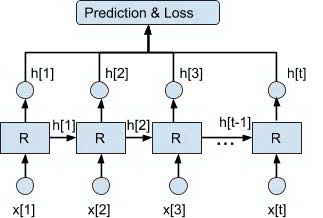$P\bigl(y|f(h[t-1],x[t])\bigr)$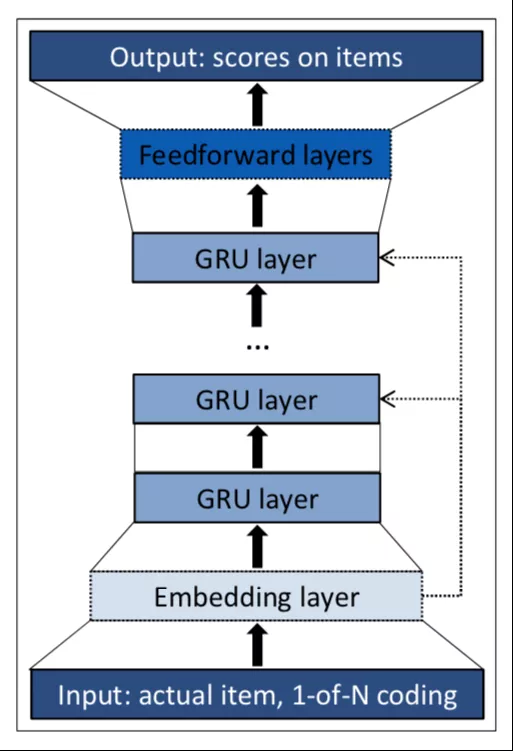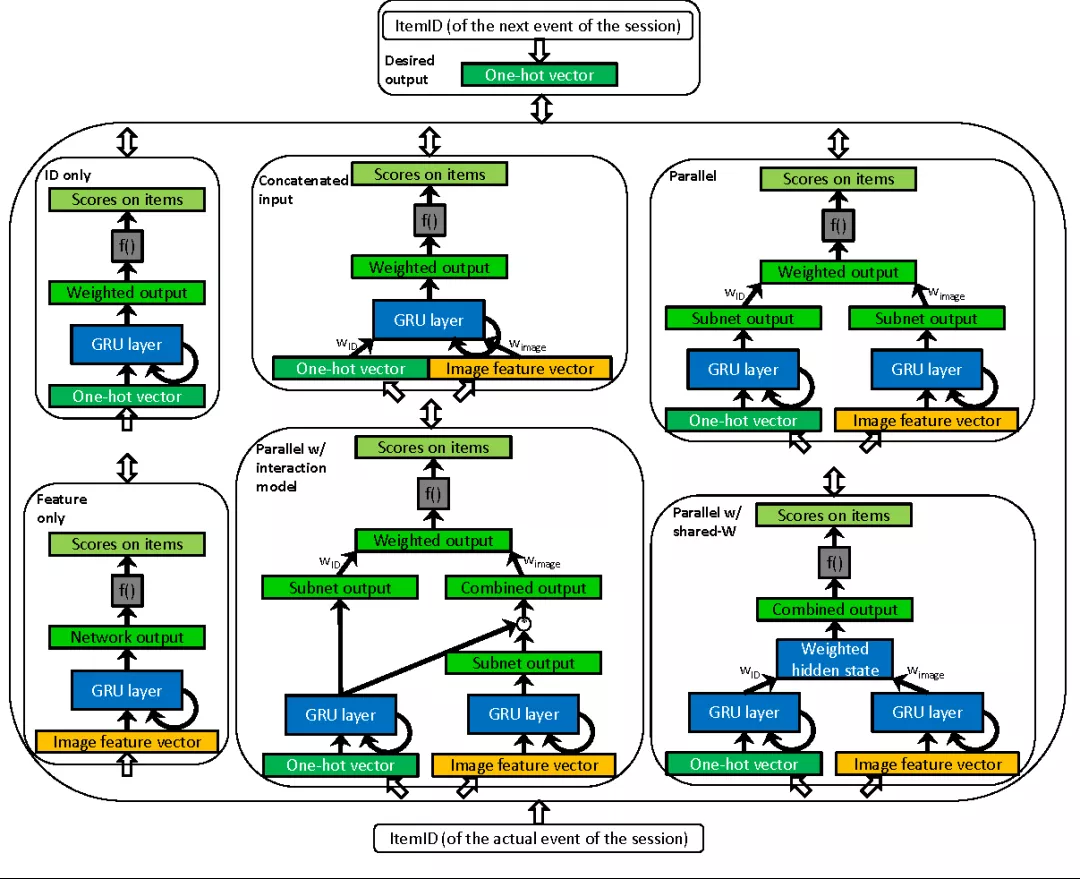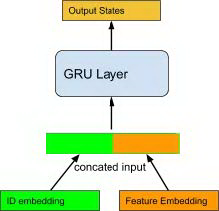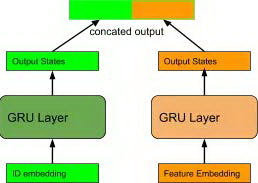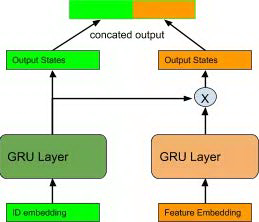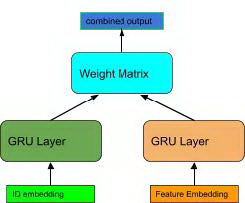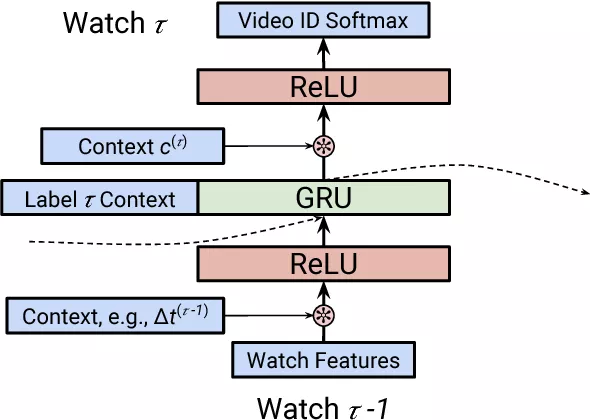1. τ -1 步用户观看视频的物品 feature 和上下文 feature c<τ-1>，两者做 dot product 作为 GRU 层的输入
2. τ 步的上下文信息 _c_<τ>，与 GRU 的输出状态进行点积，作为 softmax 的输入。文中使用到的上下文信息包括时间，设备，访问页面。上下文信息具体加入的公式是：

$h=\Bigl(1+\sum_{\tau}c_{\tau}\Bigr)*h$

## Temporal CNN

RNN 结构用于序列建模的优点是结构简单，训练和预测的资源占用小，缺点是随着序列变长容易出现梯度消失的问题，同时 RNN 的训练不容易实现并行。前面我们谈了如何在时间序列上运用 CNN 进行输入序列建模，CNN 结构通过矩阵运算的并行性容易实现模型训练的并行化，同时相对不容易出现序列变长后的梯度消失问题。因此我们希望能够像 RNN 一样在整个时间序列上通过 CNN 进行建模，这样便产生了 TCN ( TemporalCNN ) 。TCN 采用多层的一维卷积架构在序列长度的维度进行卷积，通过 zero padding 保证每一层的长度相同。TCN 架构结合了 casual convolution ( 因果卷积 )，residual connection ( 残差连接 ) 以及 dilated convolution ( 空洞卷积 )，其特点包括：

1. 采用因果卷积，保证训练过程中未来的信息不会泄露到过去时间的建模中；
2. 将任意长度的序列如同 RNN 那样映射为相同长度的输出序列。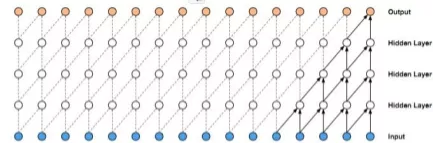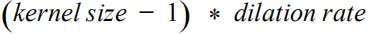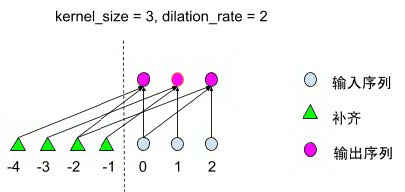def conv_with_padding(input_sequence, kernel_size, dilation_rate, output_filters):     padding_size = (kernel_size - 1) * dilation_rate     #在序列的左边补齐     padded_sequence = tf.pad(input_sequence, [[0,0], [padding_size,0], [0,0]])     conv_output = tf.layers.conv1d(padded_sequence, filters=output_filters,                                                       kernel_size=kernel_size, activation=None,                                                       padding=’VALID’, strides=1,                                                       dilated_rate=dilation_rate)     return conv_output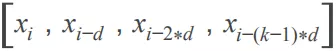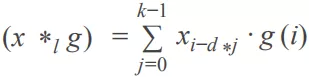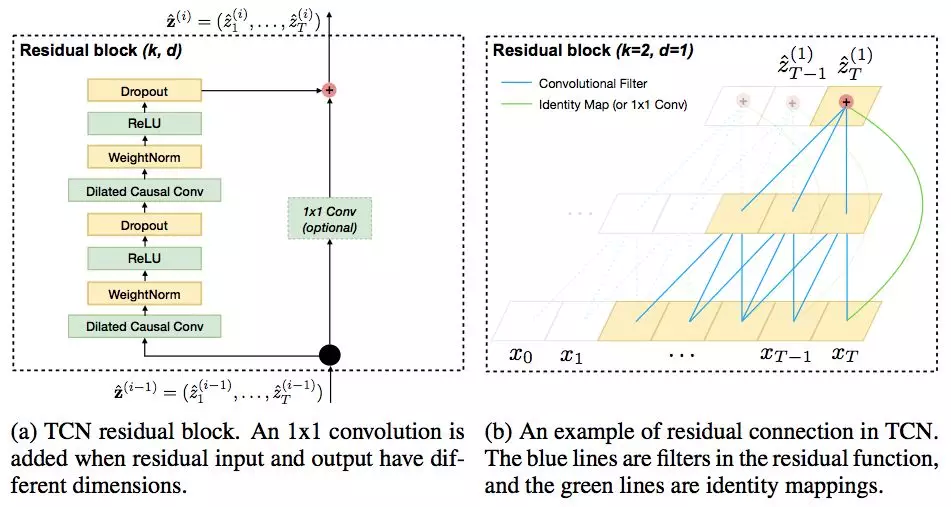1. 所有层之间实现权值共享；
2. 整个网络的输入序列作为每层输入的一部分。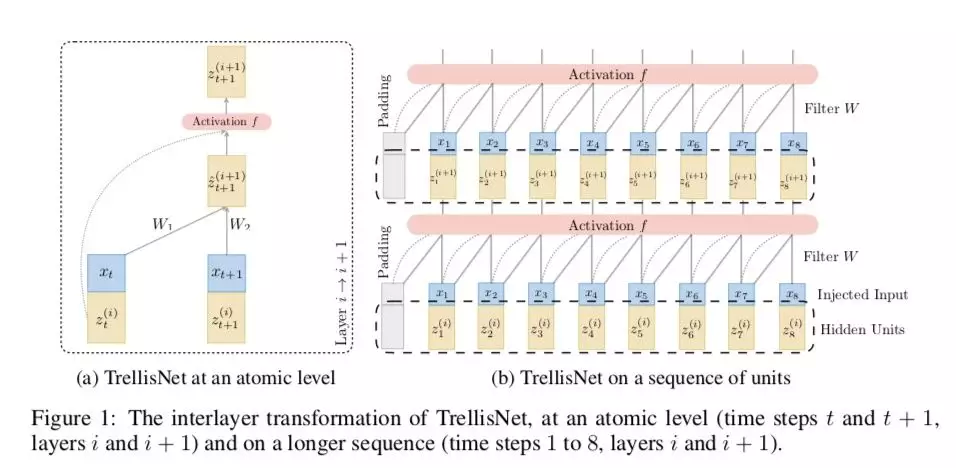TrellisNet 与 RNN 和 CNN 具有紧密的联系，因此 Trellis Network 上也可以运用 CNN 和 RNN 的某些建模技巧，例如：CNN 的大卷积核，空洞卷积，RNN 的 gated unit。关于其详细描述与证明请参见论文 。

## Self-Attention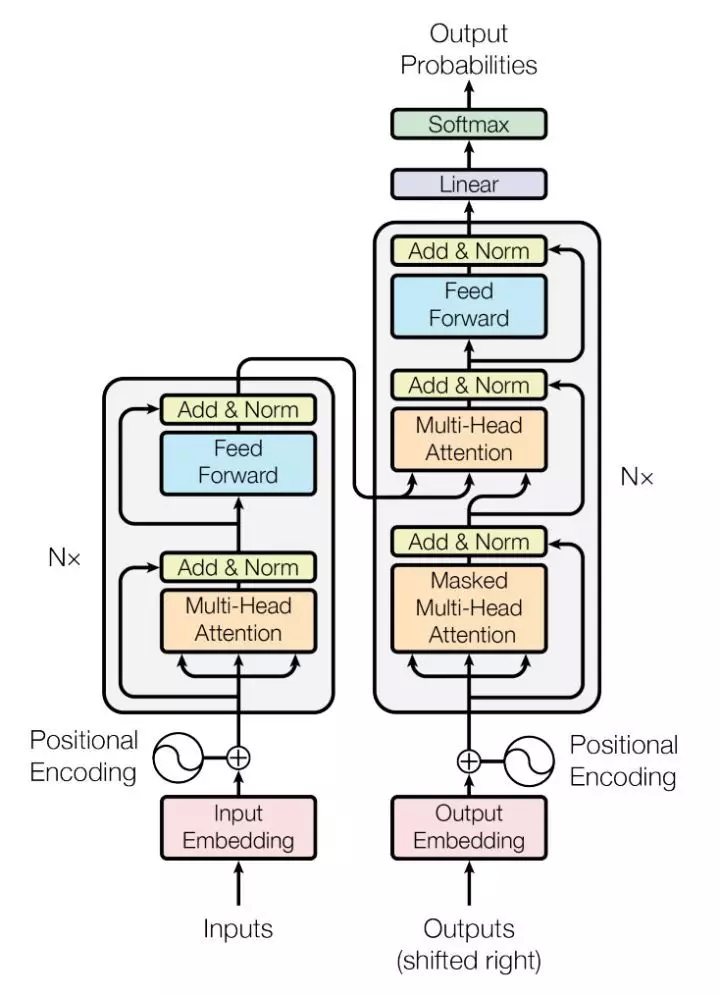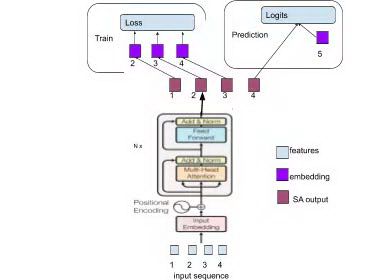Position Embedding

Self-attention Blocks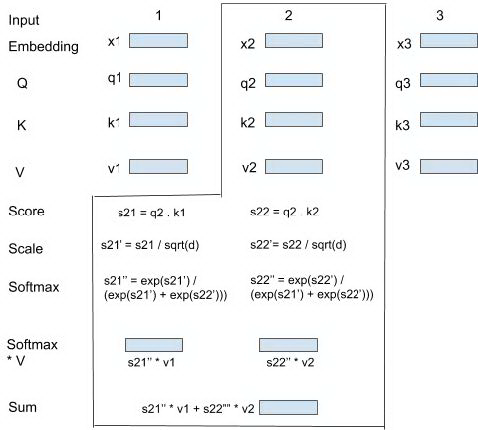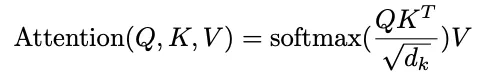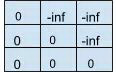M[b, i, j] = 0，表示 i 可以与 j 做 attention，M[b, i, j] = -inf ( 一个很大的负数，eg . -1e9 )，表示 i 不能与 j 做 attention。设置一个很大的负数，$QK^T/\sqrt{d_k}$ + M 经过 softmax 后不能做 attention 的位置则变为 0。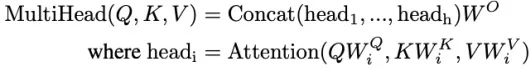② Position-wise Feed Forward

Multi-Head Attention 的输出作为 Position-wise Feed-Forward Network 的输入。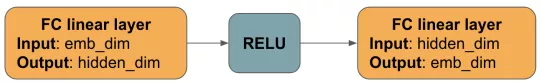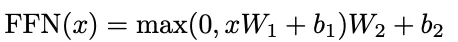③ LayerNorm & Residual Connection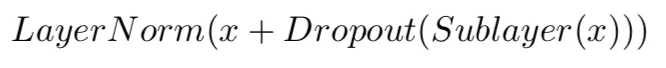Train

$R[b][t]=E[b][t]\odot O[b][t-1]$

R[b][t] 表示第 b 个序列中第 t 个时间步行为物品 ( positive item ) 的向量与 t 时刻之前位置的输出向量的相关度 ( 取 t-1 时刻来代表 t 时刻之前位置的信息 )，相当于是将 O 右移了一位，以保证我们用每个时刻之前的信息来预测在该时刻与行为物品的相关度，如图 27 中我们使用步骤 1,2,3 的输出状态去预测步骤 2,3,4。在 t 时刻我们可以随机 sample negative items 然后采用 pairwise rank loss，增大 postivie item 的相关度并减小 negative item 的相关度。

Prediction

predict_score = $V\odot W$

## 强化学习

$P[S_{t+1}|S_t]=P[S_{t+1}|S_1,\dots,S_t]$

agent 推荐物品给用户之后，根据用户对推荐列表的反馈 ( 忽略或者点击 ) 来得到 ( 状态 - 行为 ) 的即时奖励 reward：

$E[R_{t+1}|S_t=s,A_t=a]$

$R_{t+1}$ 中 t+1 表示奖励具有延迟性，即在一个时刻发生行为，在下一个时刻收到环境的反馈。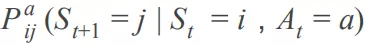$G_t=R_{t+1}+\gamma R_{t+2}+\dots=\sum^{\infty}{k=0}\gamma^kR{t+k+1}$

$\pi(a|s)=P(A_t=a|S_t=s)$

$v_{\pi}(s)=E_{\pi}[R_t|S_t=s]$

$q_{\pi}(s,a)=E_{\pi}[R_t|S_t=s,A_t=a]$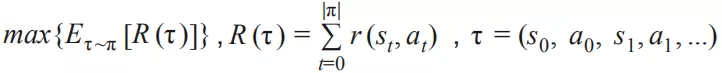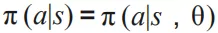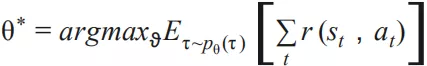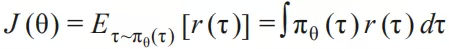θ 求导：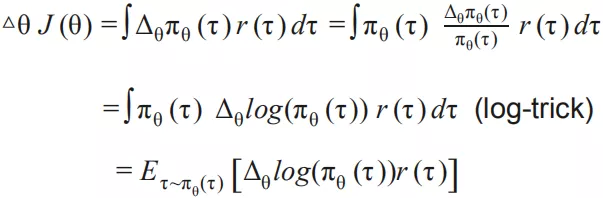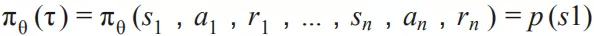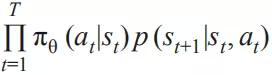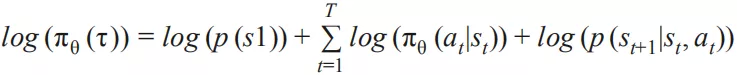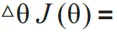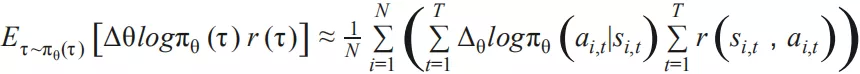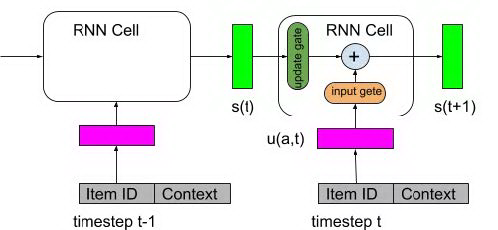$s(t+1)=f(s(t),u(a,t))=z(t)\odot s(t)+i(t)\odot tanh\bigl(W_au(a,t)\bigr)$

$\pi_{\theta}(a|s)=exp\bigl(s(t)v(a)/T\bigr)/\sum_{a’}exp\bigl(s(t)v(a’)/T\bigr)$

v(a) 是候选物品的向量，上面这个公式即 youtube DNN 做召回的思想：在实际使用中，用 RNN 的输出状态做 k-nearest neighbor search， 得到一个相对较小的候选集合，然后再在这个候选集合中计算每一个候选物品的概率。

## 总结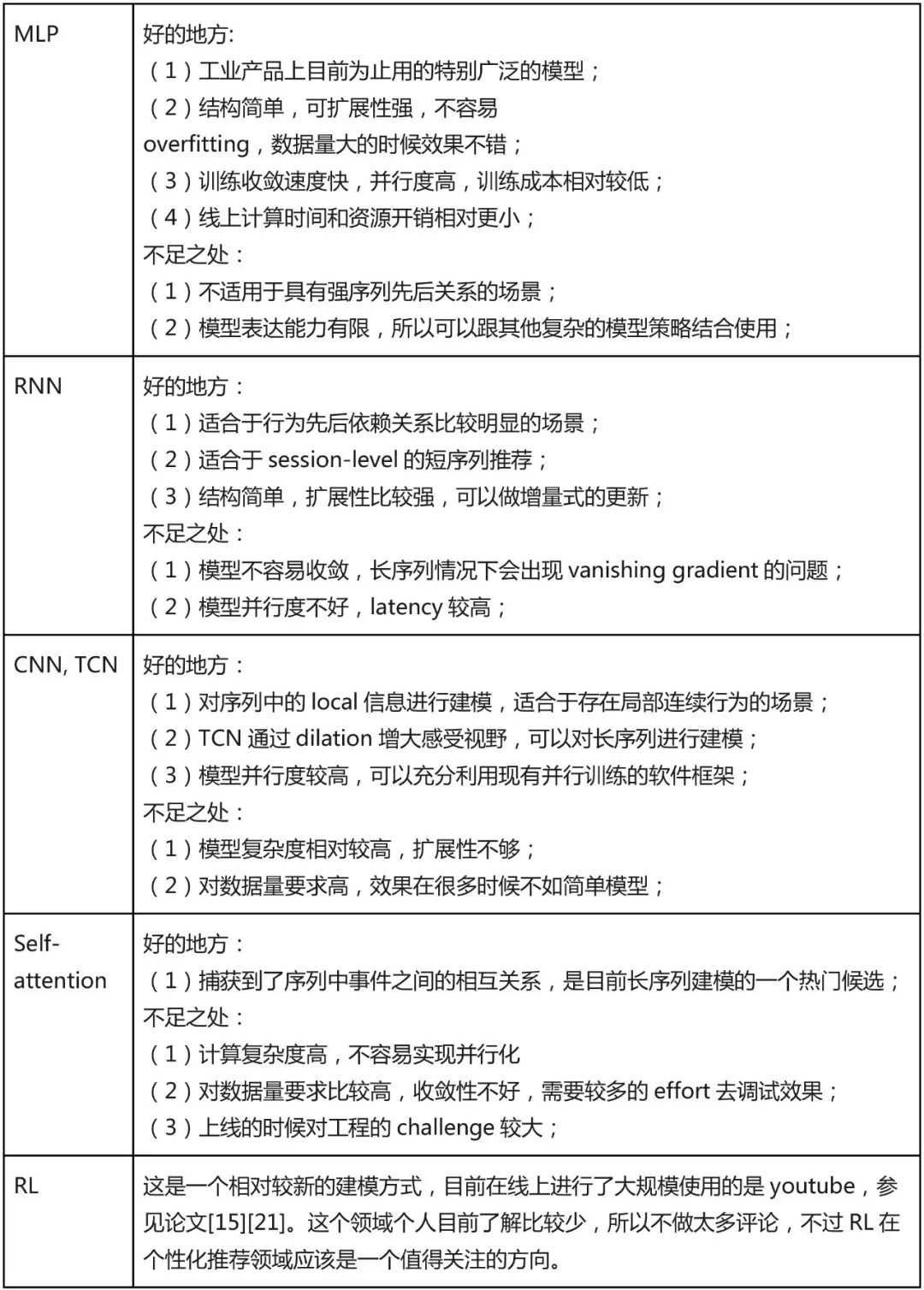1. 找适合业务需求的：根据自身的业务目标，当前的数据量，数据中信息丰富程度，工程架构上的支持，训练成本，上线成本等来折中考虑。
2. 先选简单的，简单的模型大部分情况下都 work 的比较好。

## 参考文献

 Deep Learning based Recommender System: A Survey and New Perspectives

 Deep learning sequential recommendation systems

 Image Matters: Visually modeling user behaviors using Advanced Model Server

 Deep Interest Network for Click-Through Rate Prediction

 Convolutional Sequence Embedding Recommendation Model

 Convolutional Neural Networks for Sentence Classification

 Session-based recommendations with recurrent neural networks

 Parallel Recurrent Neural Network Architectures for Feature-rich Session-based Recommendations

 Hierarchical question-image co-attention for visual question answering

 An Empirical Evaluation of Generic Convolutional and Recurrent Networks for Sequence Modeling

 Trellis Networks for Sequence Modeling

 Attention Is All You Need

 Layer Normalization

 Deep Neural Networks for YouTube Recommendations

 Top-K Off-Policy Correction for a REINFORCE Recommender System

 Latent Cross: Making Use of Context in Recurrent Recommender Systems

 Convolutional sequence modeling revisited

 Learning Deep Structured Semantic Models for Web Search using Clickthrough Data

 ATRank: An Attention-Based User Behavior Modeling Framework for Recommendation